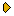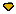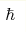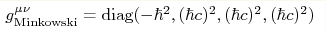HomeFor authorsSubmission statusCurrentArchive (English)ArchiveVolumes 61-80Volumes 41-60Volumes 21-40Volumes 1-20Volumes 81-92 Volume 92 Volume 91 Volume 90 Volume 89 Volume 88 Volume 87 Volume 86 Volume 85 Volume 84 Volume 83 Volume 82 Volume 81SearchVOLUME 90 (2009) | ISSUE 11 | PAGE 793as parameter of Minkowski metric in effective theory
PACS: 03.65.-w, 04.20.-q, 05.20.Jr
Abstract
With the proper choice of the dimensionality of the metric components and matter field variables, the action for all fields becomes dimensionless. Such quantities as the vacuum speed of light c, the Planck constant, the electric charge e, the particle mass m, the Newton constant G never enter equations written in the covariant form, i.e., via the metric gμν. The speed of light c and the Planck constantare parameters of a particular two-parametric family of solutions of general relativity equations describing the flat isotropic Minkowski vacuum in effective theory emerging at low energy:. They parametrize the equilibrium quantum vacuum state. The physical quantities which enter the covariant equations are dimensionless quantities and quantities which have dimension of rest energy M or its power. Dimensionless quantities include the running coupling `constants' αi; the geometric θ-parameters which enter topological terms in action; and geometric charges coming from the group theory, such as angular momentum quantum number j, weak charge, electric charge q, hypercharge, baryonic and leptonic charges, number of atoms N, etc. Dimensionful parameters are mass matrices with dimension of M; gravitational coupling K with [K]=[M]2; cosmological constant with dimension M4; etc. In effective theory, the interval s has the dimension of 1/M; it characterizes dynamics of particles in quantum vacuum rather than space-time geometry. The action is dimensionless reflecting equivalence between action and the phase of a wave function in quantum mechanics. We discuss the effective action, and the measured physical quantities including parameters of metrology triangle.